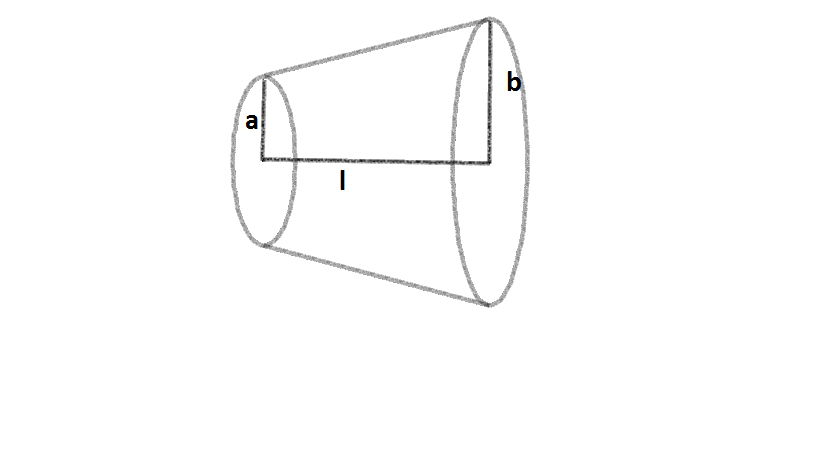# I'm gonna make him an offer he can't resist(ance)A conductor of length $l$ has a uniform cross section.

The radius of cross section varies linearly from $a$ to $b$.The resistivity of the material is $\rho$.

Find the resistance of the conductor across its ends in Ohms.

Details and Assumptions

• $\rho$=$6.28\times$$10^{-8}$$\Omega$m
• $l$ (length of conductor) =10 cm
• $a$=2 cm and $b$=5 cm
×

Problem Loading...

Note Loading...

Set Loading...